# Deriving equation of wave motion

• Pushoam

#### Pushoam

The equation below (2.9) is also a linear differential equation.
This equation also describes the wave phenomena.
So, why is this equation not considered as wave equation?
I have taken it from the optics book by Chapter two Eugene Hecht,5th edition ,Pearson.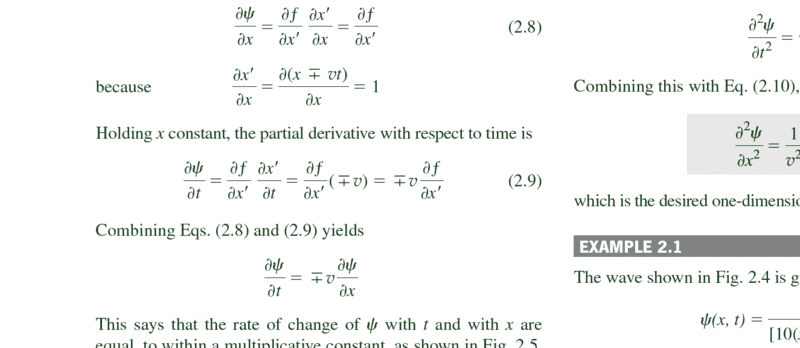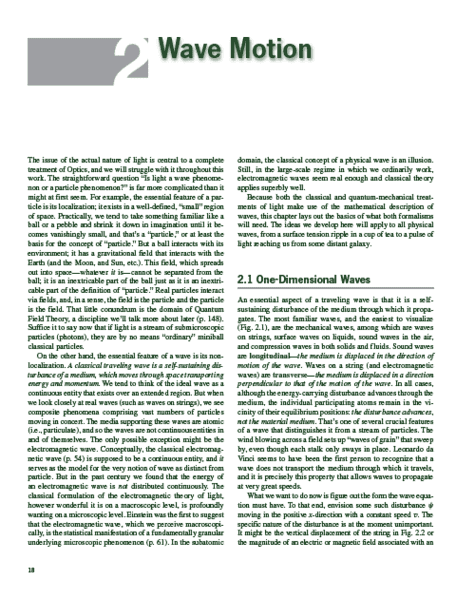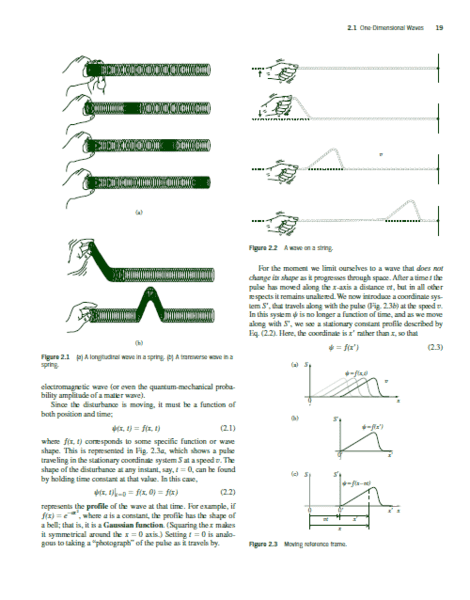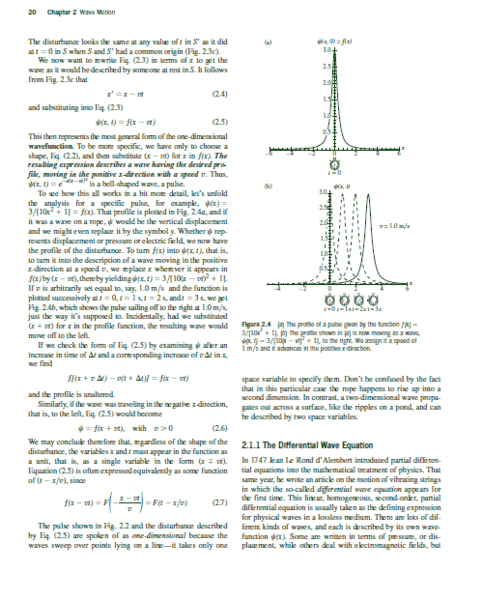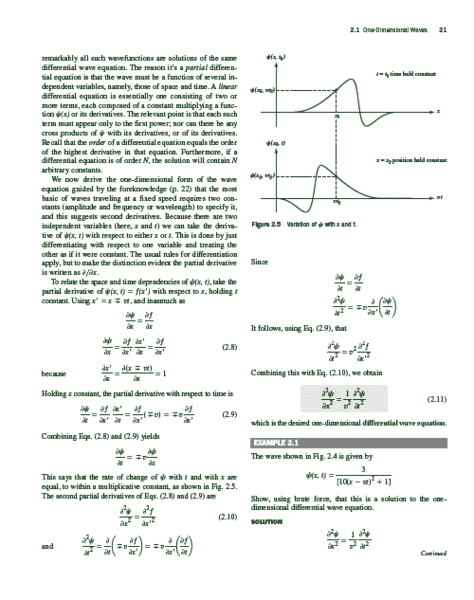Last edited:
I must say that this is the worst derivation of the D'Alambert equation I've ever seen. It's simple but seems to describe any differentiable function. It is true that "wave" is a really general concept, in fact there are many different kind of waves and many different equation describing them, but it's not like anything is a wave!
Anyway, as the text say on page 21, you need at least a second order differential equation to describe a wave since it has in the simplest case an amplitude and a frequency, so two parameters which requires two conditions.